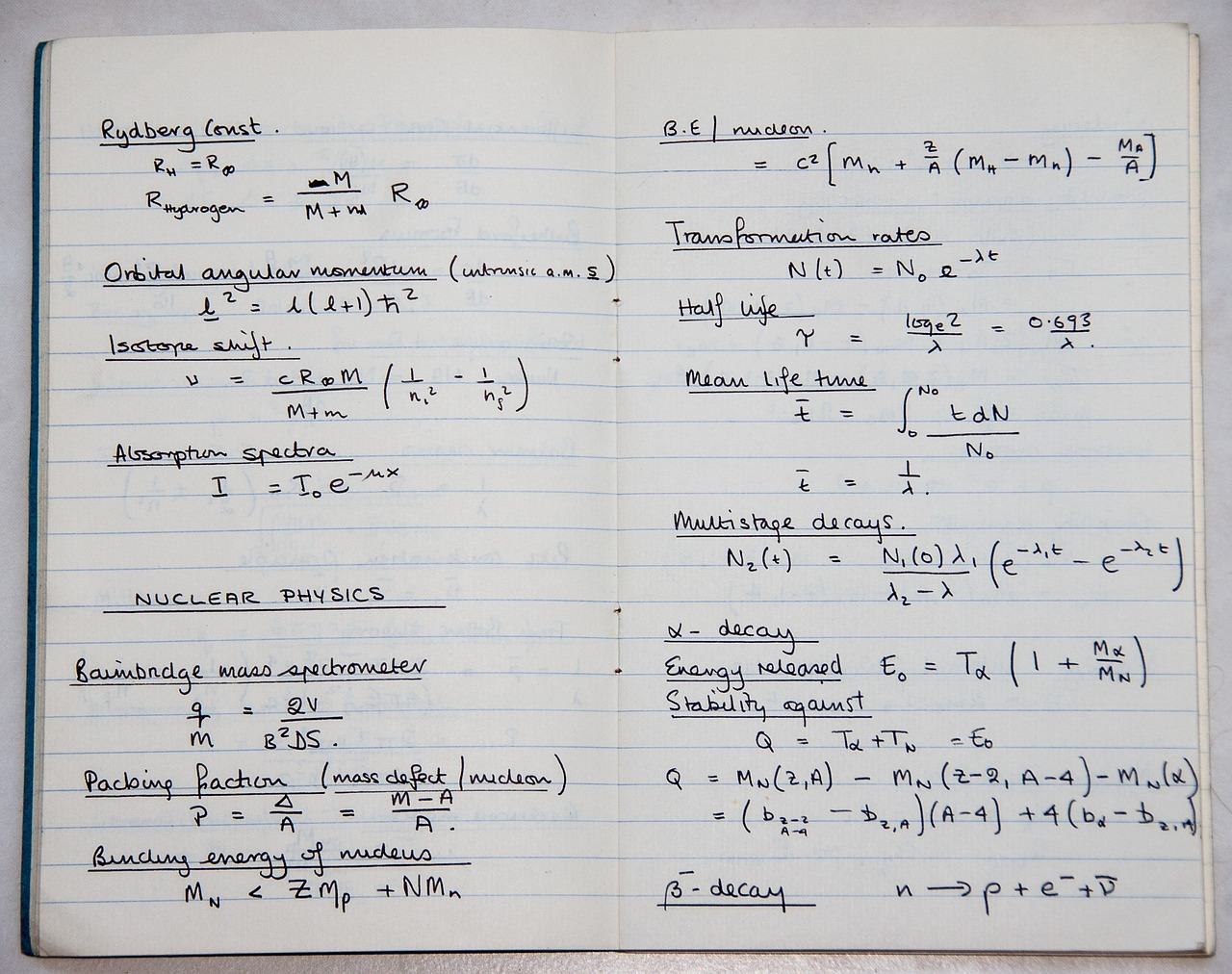# What Is Statistical Significance?

In our preliminary data on homeschool child fatalities, we stated that while the number of homeschool fatalities is larger than we would expect for the size of the homeschool population, the difference is not yet “statistically significant.” (If you’re interested in reading the full breakdown of the numbers, see child abuse fatalities, ages 6-17.) Some have asked what we mean by statistically significant, so we’ve written up this piece to explain.

When we look at the number of school aged children in the general population who were killed by their parents or guardians during a given year, we can multiply that number by the percentage of children homeschooled to get the predicted number of homeschool fatalities we would see that year if the rate were the same. For example, if there were 300 child fatalities among school aged children in a given year, and 3% of children were homeschooled that year, we could predict that there would be 9 homeschool fatalities.

However, the numbers we are working with are fairly small, and while we would expect the numbers to average out over the long term, we would also expect to see some variation in a given year. For example, one year we might see 12 fatalities, and the next year 7 fatalities, and so on. If you flipped a coin four times, you wouldn’t necessarily get two heads and two tails. But if you flipped a coin four times, wrote the result, and then did the same thing twenty more times, you would expect the total number of heads and tails to even out even as the results of the individual batches of four might vary. In other words, some random variation is normal.

According to our preliminary data, we would predict 73 homeschool fatalities during the years we examined based on the overall number of fatalities and the percentage of children homeschooled, but we have in fact catalogued 84 homeschool fatalities. Obviously, the number 84 is larger than the number 73, meaning that we have found more homeschool fatalities than we would have expected given the percentage of children homeschooled. However, there is some chance that this difference is simply random.

If we flipped a coin 100 times and got 48 heads and 52 tails, there is a very good chance that the difference is simply random. However, if we flipped the same coin 100 times and got 30 heads and 70 tails, we would suspect that the coin may be weighted. In the same way, there is some chance that our fatality number (84) is higher than the predicted number (73) as a result of random chance rather than as a result of any factor specific to homeschooling.

“Statistical significance” is a way statisticians differentiate between results that could be the product of randomness and results that are likely not the result of randomness. We ran the numbers and found that, given the relative size of the numbers, our results do not yet meet the standards for statistical significance. This means that there is a chance that the difference between the two numbers could be the result of randomness rather than the result of any factor related to homeschooling. As we have noted, we anticipate the number of homeschool fatalities we have documented to increase as our data becomes more complete, and in the process we expect that we will reach statistical significance. As it is, we can say for certain that the rate of homeschool fatalities is at least not lower than the rate of school age fatalities in the nation as a whole.